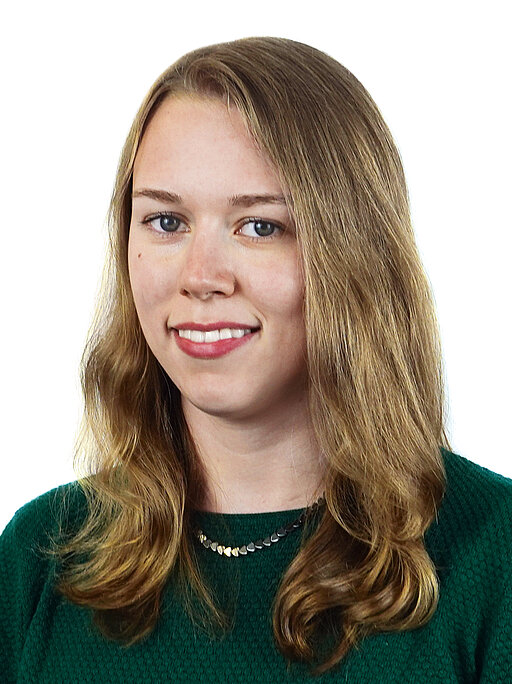•# Kathrin Hellmuth

### Kathrin Hellmuth

Chair of Mathematics VI
Emil-Fischer-Straße 40
97074 Würzburg
Building: 40 (Mathematik Ost)
Room: 03.015Conference proceedings:

Science communication:

Various natural phenomena can be described using partial differential equations (PDEs). One example is the movement of bacteria stimulated by a chemical signal, which is called chemotaxis. It is often modelled by a kinetic chemotaxis equation or a macroscopic Keller Segel equation and there exists a relation between both PDE models.

In order fit the mathematical models to reality, experiments have to be conducted in order to determine the model coefficients. For chemotaxis, this means observing the bacterial motion so to reconstruct coefficients encoding the bacterial reaction to the chemical substance. I study this inverse problem, in particulat the relation between the inverse problems for the macroscopic and the kinetic model.

 Winter term 2022/23 Exercise sessions in Mathematics for Machine Learning (with Prof. Dr. Christian Klingenberg) Winter term 2021/22 Exercise sessions in Partial Differential Equations in mathematical Physics (with Prof. Dr. Christian Klingenberg) Summer term 2021 Exercise sessions in Linear Algebra 1 (with Prof. Dr. Komla Domelevo) Winter term 2020/21 Exercise sessions in Linearen Algebra 1 (with Prof. Dr. Sergey Dashkovskiy and Sandra Warnecke) Winter term 2019/20 Student teacher in Analysis 1 Tutor for first year students (JIM-Erklärhiwi)

 Oct. 2014 - Apr. 2020 Study of Mathematics, University of Wuerzburg, Germany Erasmus+ semester at the university of Padua, Italy, in summer 2018 Apr. 2020 Master degree Master thesis: Computing the Black Scholes equation with uncertain volatility                          using the stochastic Galerkin method and a Bi-Fidelity approach Advisor: Prof. Dr. Christian Klingenberg Mar. 2020 - now PhD studies, Institute of Mathematics, University of Wuerzburg, Germany Advisor: Prof. Dr. Christian Klingenberg